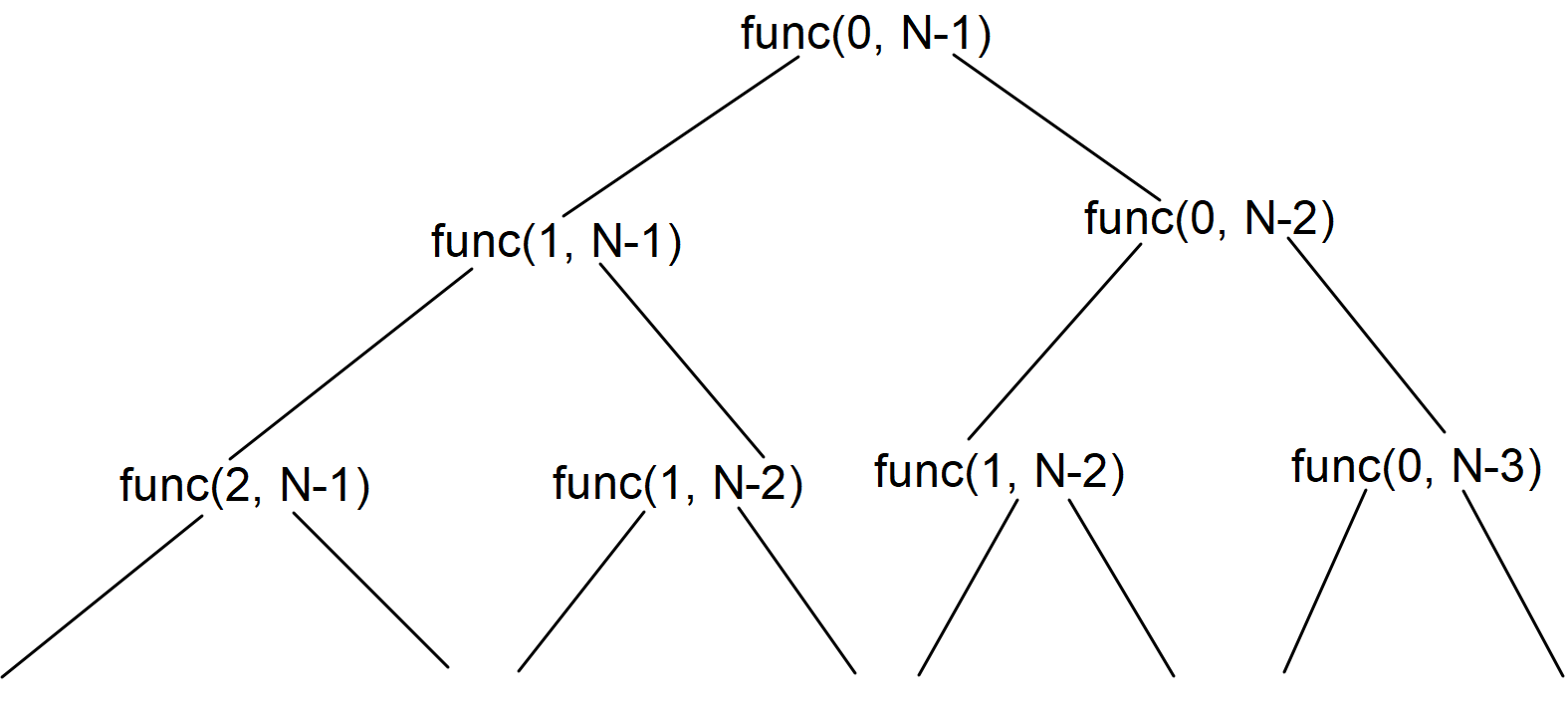# Minimum insertion to convert the string to a palindrome

## Introduction:

We are given a string. We need to find the minimum number of characters we need to add to the string to convert the given string to a palindrome string.

Let us see a few examples.

Input:

“abc”

Output:

2

Explanation:

We need to add ‘b’ and ‘a’ at the end of the String(“abcba”) to make it a palindrome string.

Input:

“aba”

Output:

0

Explanation:

The input string is already a palindrome string. Hence, 0 insertions are needed to make it Palindrome.

## Approach 1:

We can use a recursive approach here. We will keep two pointers ‘i’ and ‘j’. ‘i’ will be at the 0th index, and the ‘j’ pointer will be at the (N - 1)th index.

If the characters at the ‘i’ and ‘j’ positions are equal, the answer would be calculated by calling the recursive function (i + 1, j - 1).

Also, we will call the recursive function for fun(i + 1, j) + 1 and fun(i, j - 1) + 1. We are adding 1 to the answer of both the recursive calls because we need to add one character to balance the ‘i’th or ‘j’th character. In the end, we will return the minimum among them.

Refer to the below implementation of the above approach.

Time Complexity: The time complexity of the above approach is O(2 ^ N). In the worst case, we will be trying both the cases of either incrementing the ‘i’ pointer or decrementing the ‘j’ pointer.

Space Complexity: The space complexity of the above approach is O(1) because we are using the constant auxiliary space.

## Approach 2:

If we observe the above approach, we can find that it exhibits overlapping subproblems.In the above recursion tree, we can see that we are calculating func(1, N - 2) twice.

Hence, we can use dynamic programming to solve this question efficiently. We can store the minimum number of insertions needed to make str[i...j] palindrome for all the (i, j) pairs and use them whenever we need them again. We will follow the gap strategy to solve this question.

We will first calculate the answer for the substrings of length 1. Then using the answer of the length 1 substrings, we will calculate the answer for the 2 length substrings. In a similar way, we will keep calculating the answer for all the substrings.

Suppose we have a String of length 5. We will be calculating the answer in the following order.

First we will calculate answer for gap = 1:-

Gap = 1: (0, 1) (1, 2) (2, 3) (3, 4)

We will calculate answer for gap = 2:-

Gap = 2: (0, 2) (1, 3) (2, 4)

We will calculate answer for gap = 3:-

Gap = 3: (0, 3) (1, 4)

We will calculate answer for gap = 4:-

Gap = 4: (0, 4)

Refer to the below implementation of the above approach.

Time Complexity: The time complexity of the above approach is O(N * N) because we are running two nested for loops of O(N) time each.

Space Complexity: The space complexity of the above approach is O(N * N) because we are maintaining a D.P. table of size O(N * N).

## Approach 3:

We can also solve this question using the concept of L.C.S.(Longest common subsequence). We need to find the minimum insertions to make the string a palindrome. If we find the longest palindromic subsequence in the String, we can balance the rest of the characters which are not part of the longest palindromic subsequence using insertions.

To find the longest palindromic subsequence of the given string we can find the L.C.S. of the input String and the reversed input string.

Let ‘L’ be the length of the longest palindromic subsequence of the input string and ‘length’ be the length of the entire input string.

The minimum number of insertions to make the entire String palindrome is (length - L).

You can refer to the following blog for finding the longest common subsequence.

## FAQs:

1. What optimization did we do on the brute force approach to solve this problem?
• We saw that in approach 1 there were overlapping subproblems. Hence, we used the D.P. table to store the answer for different (i, j) pairs to avoid calculating the answer for an already computed (i, j) pair.

1. What is the time complexity for the optimized approach?
• The time complexity for the optimized approach-2 is O(N*N) because we are running two nested for loops of O(N) time each.

## Key Takeaways:

In this blog, we have covered the following things:

1. We first discussed the Brute Force approach to solve this problem.
2. Then we saw how we optimized the brute force approach by applying Dynamic Programming.

If you want to learn more about Dynamic Programming and want to practice some questions which require you to take your basic knowledge on Dynamic Programming a notch higher, then you can visit our Guided Path for Dynamic Programming. To practice this problem, you can visit Practice Problem.

Until then, All the best for your future endeavors, and Keep Coding.